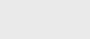3年前 (2020-10-12) |   抢沙发  563

## 递归算法示例

### 布朗桥

``````public class Brownian {

// midpoint displacement method
public static void curve(double x0, double y0, double x1, double y1, double var, double s) {
// stop if interval is sufficiently small
if (Math.abs(x1 - x0) < 0.01) {
StdDraw.line(x0, y0, x1, y1);
return;
}

double xm = (x0 + x1) / 2;
double ym = (y0 + y1) / 2;
ym = ym + StdRandom.gaussian(0, Math.sqrt(var));
curve(x0, y0, xm, ym, var/s, s);
curve(xm, ym, x1, y1, var/s, s);
}

public static void main(String[] args) {
double hurstExponent = Double.parseDouble(args);
double s = Math.pow(2, 2*hurstExponent);
curve(0.0, 0.5, 1.0, 0.5, 0.01, s);
}
} ``````

• 计算区间的中点`（xm，ym）`
• 在中点的y坐标`ym`上加上一个随机值`δ`，从平均值为0和给定方差的高斯分布中提取。
• 在子区间上重复，将方差除以给定的比例因子s。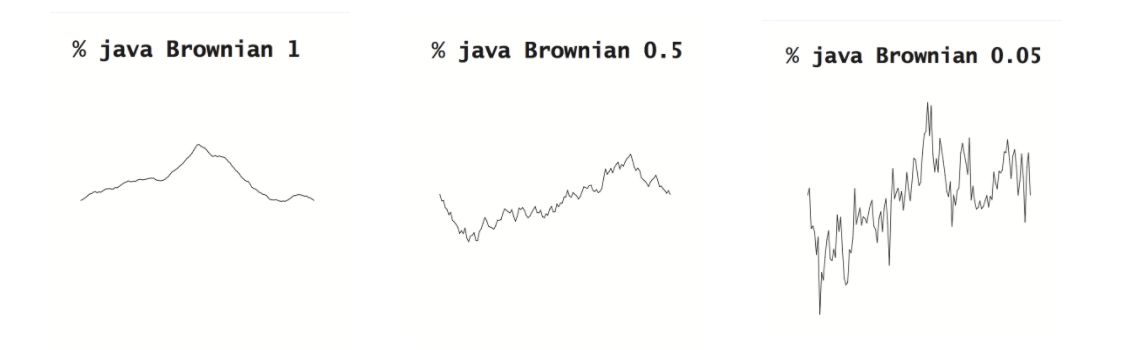### 递归的陷阱

1.缺少基本情况

``````public class NoBaseCase {

public static double harmonic(int n) {
return harmonic(n-1) + 1.0/n;
}

public static void main(String[] args) {
int n = Integer.parseInt(args);
StdOut.println(harmonic(n));
}

}``````

2.不能保证趋同

``````public class NoConvergence {

public static double harmonic(int n) {
if (n == 1) return 1.0;
return harmonic(n) + 1.0/n;
}

public static void main(String[] args) {
int n = Integer.parseInt(args);
StdOut.println(harmonic(n));
}

}
``````

3.内存需求过大

``````public class ExcessiveMemory {

public static double harmonic(int n) {
if (n == 1) return 1.0;
return harmonic(n-1) + 1.0/n;
}

public static void main(String[] args) {
int n = Integer.parseInt(args);
StdOut.println(harmonic(n));
}

}
``````

4.过度的重新计算

``0，1，1，2，3，5，8，13，21，34，55，89，144，233。。。``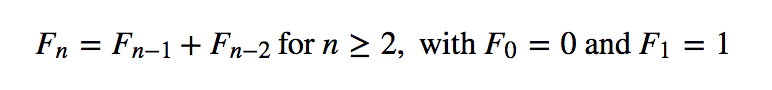``````public class Fibonacci {
public static long fibonacci(int n) {
if (n <= 1) return n;
else return fibonacci(n-1) + fibonacci(n-2);
}

public static void main(String[] args) {
int n = Integer.parseInt(args);
for (int i = 1; i <= n; i++)
StdOut.println(i + ": " + fibonacci(i));
}

}``````

### 动态规划

``````public class TopDownFibonacci {
private static long[] f = new long;

public static long fibonacci(int n) {
if (n == 0) return 0;
if (n == 1) return 1;

// return cached value (if previously computed)
if (f[n] > 0) return f[n];

// compute and cache value
f[n] = fibonacci(n-1) + fibonacci(n-2);
return f[n];
}

public static void main(String[] args) {
int n = Integer.parseInt(args);
for (int i = 1; i <= n; i++)
StdOut.println(i + ": " + fibonacci(i));
}

}``````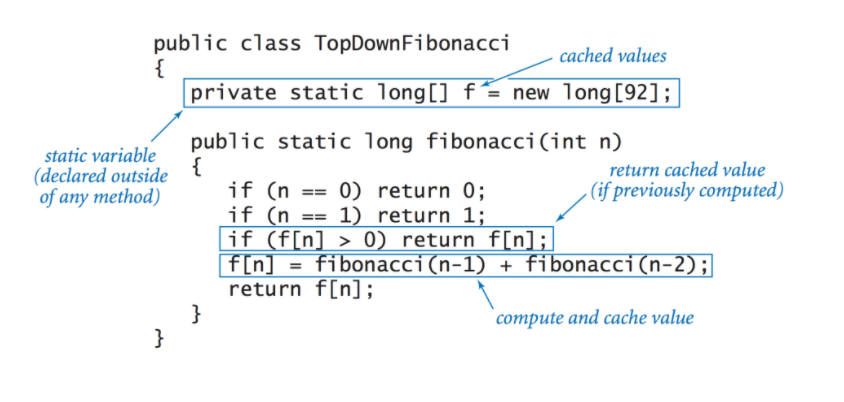1.自下而上的动态规划

``````public class BottomUpFibonacci {

public static long fibonacci(int n) {
long[] f = new long[n+1];
f = 0;
f = 1;
for (int i = 2; i <= n; i++)
f[i] = f[i-1] + f[i-2];
return f[n];
}

public static void main(String[] args) {
int n = Integer.parseInt(args);
for (int i = 1; i <= n; i++)
StdOut.println(i + ": " + fibonacci(i));
}

}``````

2.最长公共子序列问题

``````- - G G C - - A - C C A C G
A C G G C G G A T - - A C G``````

3.最长公共子序列递归

``````opt[i][j] = 0                              if i = m or j = n
= opt[i+1][j+1] + 1              if s[i] = t[j]
= max(opt[i][j+1], opt[i+1][j])  otherwise``````

4.动态规划解决方案

``````public class LongestCommonSubsequence {

// Compute length of LCS for all subproblems.
public static String lcs(String x, String y) {
int m = x.length(), n = y.length();
int[][] opt = new int[m+1][n+1];
for (int i = m-1; i >= 0; i--) {
for (int j = n-1; j >= 0; j--) {
if (x.charAt(i) == y.charAt(j)) {
opt[i][j] = opt[i+1][j+1] + 1;
}
else {
opt[i][j] = Math.max(opt[i+1][j], opt[i][j+1]);
}
}
}

// Recover LCS itself.
String lcs = "";
int i = 0, j = 0;
while (i < m && j < n) {
if (x.charAt(i) == y.charAt(j)) {
lcs += x.charAt(i);
i++;
j++;
}
else if (opt[i+1][j] >= opt[i][j+1]) i++;
else                                 j++;
}
return lcs;
}

public static void main(String[] args) {
String lcs = lcs(args, args);
StdOut.println(lcs);
}
}
``````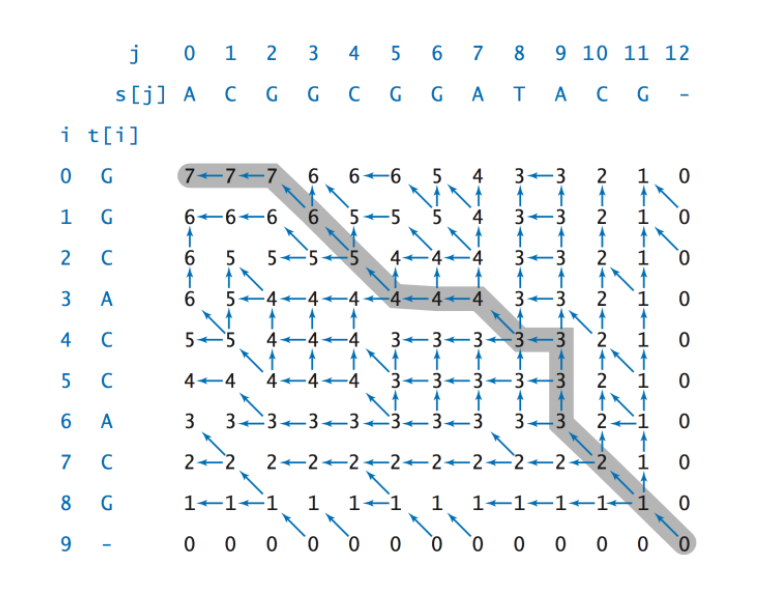• 字符`s[i]``t[j]`匹配。在这种情况下，必须有`opt[i][j]=opt[i+1][j+1]+1`，并且LCS中的下一个字符是`s[i]`。我们继续从`opt[i+1][j+1]`追溯
• LCS不包含`s[i]`。在本例中，`opt[i][j]=opt[i+1][j]`，我们继续从`opt[i+1][j]`追溯
• LCS不包含`t[j]`。在本例中，`opt[i][j]=opt[i][j+1]`，我们继续从`opt[i][j+1]`追溯### 注册